#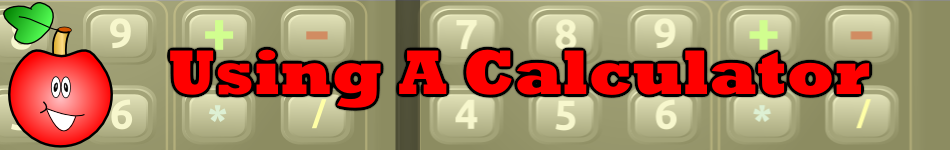There are 366 different Starters of The Day, many to choose from. You will find below some starters on the topic of Using A Calculator. A lesson starter does not have to be on the same topic as the main part of the lesson or the topic of the previous lesson. It is often very useful to revise or explore other concepts by using a starter based on a totally different area of Mathematics.

Main Page

### Using A Calculator Starters:Use only the 1, 5 and 0 keys on a calculator to make given totals.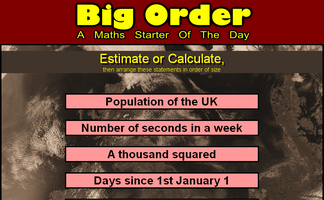Estimate or calculate then put the large numbers in order of size.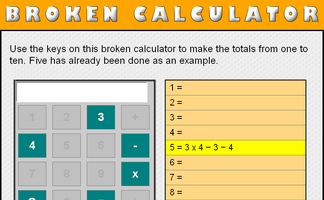Some keys are missing from this calculator. Just how useful is it?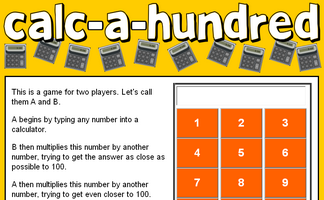A game for two players requiring a calculator and thinking skills.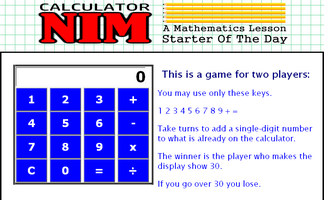A game in which players take turns to add a single-digit number to what is already in the calculator. The winner is the player who makes the display show 30.Questions about the Small LEDs used to make up the digits on a calculator display.How old is a person if when her age is divided by certain numbers, the calculator display ending are as shown.Find out which of the calculator keys is faulty from the given information. A mathematical puzzle requiring good problem solving strategies.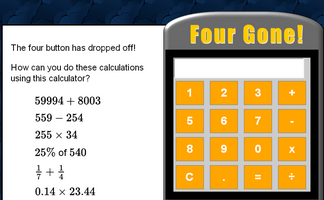An activity involving a broken calculator which is missing the four button. Can you evaluate the given expressions without using the four?Find fractions between two given values.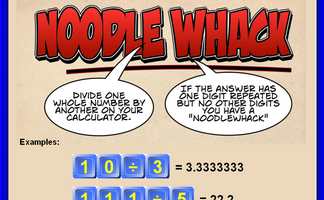Divide one whole number by another on your calculator. If the answer has one digit repeated but no other digits you have a Noodlewhack.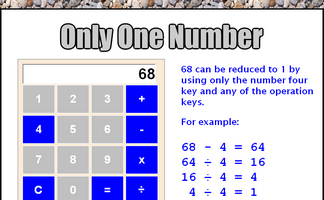Find other numbers that can be changed to 1 on a calculator using only the 4 key and any operation.Use your calculator to find which whole number divided by another whole number gives a recurring decimal.Find a calculation for the current year which uses all of the digits 1 to 9.An activity involving a calculator which is missing the six button. Can you evaluate the given expressions without using the six?Use just six calculator keys to make a total of 20. In how many different ways can it be done?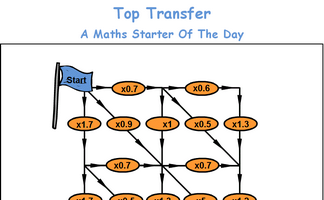Beginning with 100 on your calculator, what is the largest and smallest totals you can end up with after travelling through each of the possible routes.

## Exercises#### Animated Calculator

A visual aid calculator for projection onto a whiteboard or use in remote teaching.#### BIDMAS

A self marking exercise testing the application of BIDMAS, an acronym describing the order of operations used when evaluating expressions.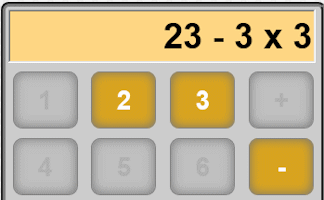#### Broken Calculator

Some of the buttons are missing from this calculator. Can you make the totals from 1 to 20?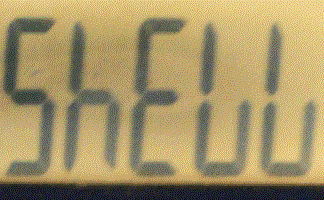#### Calculator Words

Turn your calculator upside down to make words out of the answers to these questions.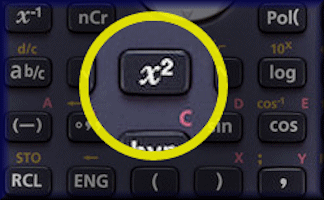#### Calculator Workout

An animated guide to using a scientific calculator for Secondary and High School students.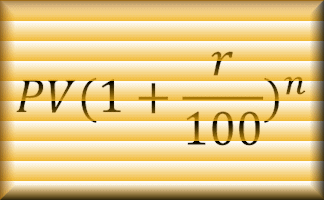#### Compound Interest Calculator

A customised online calculator for quickly finding the solutions to compound interest problems.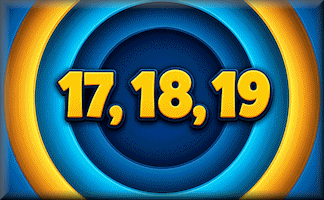#### Consecutive Numbers

Find the consective numbers that are added or multiplied to give the given totals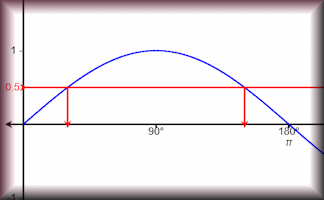#### Inverse Trig Calculator

This calculator is designed to find all of the angles for a given trigonometric ratio and show them on a graph.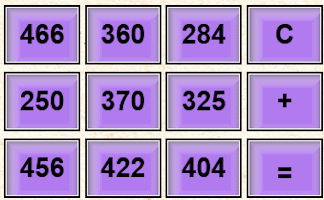#### Make 1000

Use the numbers on the strange calculator to make a total of 1000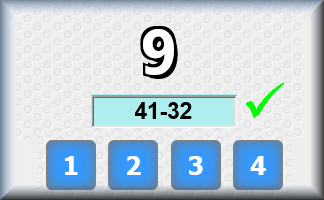#### Make an expression

Use the digits given to form an expression equivalent to the given total.#### Normal Distribution Calculator

A customised online calculator for quickly finding areas under the normal distribution curve.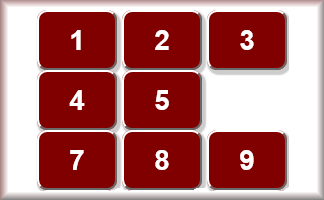#### Six Discrimination

The six button has dropped off! How could these calculations be done using this calculator?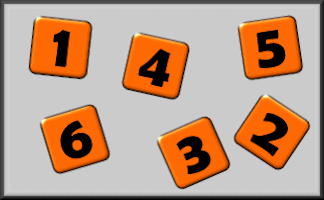#### Six Keys

Use just six keys on your calculator to make a given total. How many different ways can it be done?#### TI-Nspire Essential Skills

Learn a skill a day for 20 days in order to get the most out of this graphic display calculator.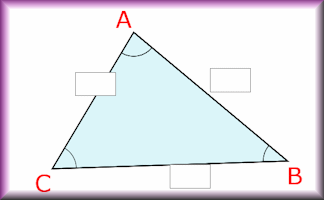#### Triangle Solver

Provide and three measurements for a triangle and this application will work out the remaining sides, angles and area.#### Using A Calculator

Questions to test your ability to use a basic calculator, scientific calculator and a graphic display calculator.

### Search

The activity you are looking for may have been classified in a different way from the way you were expecting. You can search the whole of Transum Maths by using the box below.

Have today's Starter of the Day as your default homepage. Copy the URL below then select
Tools > Internet Options (Internet Explorer) then paste the URL into the homepage field.

Set as your homepage (if you are using Internet Explorer)

Do you have any comments? It is always useful to receive feedback and helps make this free resource even more useful for those learning Mathematics anywhere in the world. Click here to enter your comments.For All: